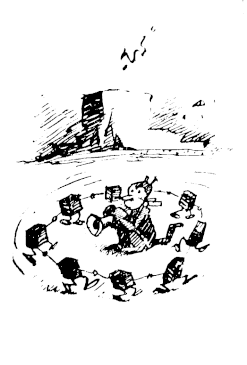The Shœstring Foundation Weblog About The Shœstring Foundation Weblog, Miscellaneous Byproducts Matthias Bauer `bauerm (at) shoestringfoundation · org ` reop pubkey Vignettes by George Herriman and a small program Subscribe to a syndicated feed of my weblog, brought to you by the wonders of RSS. Wed, 31 Oct 2018 Is the absence of bananas the same as the absence of anchovis (up to isomorphism)? This and similar hole-istic questions drove me to this: First, let's define lists and their length (in Coq): ```Inductive list' (X:Type): Type := nil : list' X cons : X -> list' X -> list' X. Arguments nil {X}. Arguments cons {X} _ _. Notation "x :: y" := (cons x y). Notation "[ ]" := nil. Notation "[ x ; .. ; y ]" := (cons x .. (cons y []) ..). Check list'. Fixpoint length' {X : Type } (l : list' X) : nat := match l with nil => O cons h t => 1 + (length' t) end. Example test_length'1 : length' ([1;2;3]) = 3. Proof. reflexivity. Qed. ``` It seems obvious that for any Type X the length of an empty list of Type X elements is zero. And indeed this is provable in Coq: ```Theorem length_type_invar: forall (X: Type), length (@nil X) = 0. Proof. intros X. simpl. reflexivity. Qed. ``` But without the quantified Type, Coq cannot check the length of an empty list: ```Example test_length'1 : length' ([]) = 0. Error: Cannot infer the implicit parameter X of nil ``` Holey Schlamoney! Two empty lists, one not containing natural numbers, the other not containing booleans, cannot be compared: ```Check @nil nat = @nil bool. Error: The term "[ ]" has type "list bool" while it is expected to have type "list nat". ``` Coq can infer the Type of an empty list: ```Example test_nil_nat: [] = @nil nat. Proof. reflexivity. Qed. Example test_nil_bool: [] = @nil bool. Proof. reflexivity. Qed. ``` So by the transitivity axiom of equality one would expect that ` [] = [] ` But an untyped nothingness cannot be compared to itself: ```Example test_nil: [] = []. Error: Cannot infer the implicit parameter X of nil whose type is "Type". ``` It is no wonder that philosophers concern themselves with the concept of holes.